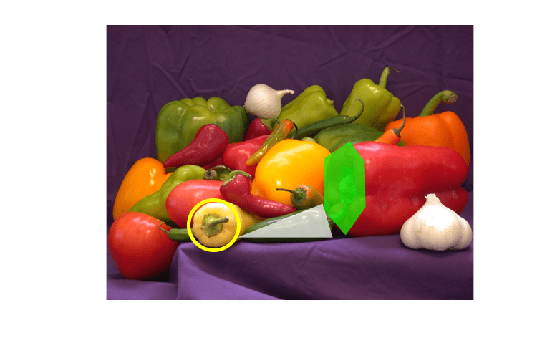# insertShape

Insert shapes in image or video

## Syntax

``RGB = insertShape(I,shape,position)``
``RGB = insertShape(___,Name,Value)``

## Description

example

````RGB = insertShape(I,shape,position)` returns a truecolor image with `shape` inserted. The input image, `I`, can be either a truecolor or grayscale image. You draw the shapes by overwriting pixel values.```

example

````RGB = insertShape(___,Name,Value)` uses additional options specified by one or more `Name,Value` pair arguments.```

## Examples

collapse all

`I = imread('peppers.png');`

Draw a circle with a border line width of 5.

`RGB = insertShape(I,'circle',[150 280 35],'LineWidth',5);`

Draw a filled triangle and a filled hexagon.

```pos_triangle = [183 297 302 250 316 297]; pos_hexagon = [340 163 305 186 303 257 334 294 362 255 361 191]; RGB = insertShape(RGB,'FilledPolygon',{pos_triangle,pos_hexagon},... 'Color', {'white','green'},'Opacity',0.7);```

Display the image.

`imshow(RGB);`## Input Arguments

collapse all

Input image, specified in truecolor or 2-D grayscale.

Data Types: `single` | `double` | `int16` | `uint8` | `uint16`

Type of shape, specified as a character vector. The vector can be, `'Rectangle'`, `'FilledRectangle'`, `'Line'`, `'Polygon'`, `'FilledPolygon'`, `'Circle'`, or `'FilledCircle'`.

Data Types: `char`

Position of shape, specified according to the type of shape, described in the table.

ShapePositionExample
`'Rectangle'`
`'FilledRectangle'`
For one or more rectangles, specify M-by-4 matrix where each row specifies a rectangle as $\left[\begin{array}{cccc}x& y& width& height\end{array}\right]$.

`$\left[\begin{array}{cccc}{x}_{1}& {y}_{1}& widt{h}_{1}& heigh{t}_{1}\\ {x}_{2}& {y}_{2}& widt{h}_{2}& heigh{t}_{2}\\ ⋮& ⋮& ⋮& ⋮\\ {x}_{M}& {y}_{M}& widt{h}_{M}& heigh{t}_{M}\end{array}\right]$`

Two rectangles, M=2`'Line'`

`'Polygon'`

`'FilledPolygon'`

For one or more disconnected lines, specify an M-by-4 matrix, where each four-element vector, $\left[{x}_{1}\text{\hspace{0.17em}}{y}_{1\text{\hspace{0.17em}}}{x}_{2\text{\hspace{0.17em}}}{y}_{2}\right]$, describes a line with endpoints.

`$\left[\begin{array}{cccc}{x}_{11}& {y}_{11}& {x}_{12}& {y}_{12}\\ {x}_{21}& {y}_{21}& {x}_{22}& {y}_{22}\\ ⋮& ⋮& ⋮& ⋮\\ {x}_{M1}& {y}_{M1}& {x}_{M2}& {x}_{M2}\end{array}\right]$`

The polyline always contains (L-1) number of segments because the first and last vertex points do not connect.

Two lines, M=2For one or more polylines or polygons with the same number of vertices, specify an M-by-2L matrix, where each row is a vector, $\left[{x}_{1}\text{\hspace{0.17em}}{y}_{1\text{\hspace{0.17em}}}{x}_{2\text{\hspace{0.17em}}}{y}_{2}\text{\hspace{0.17em}}...\text{\hspace{0.17em}}{x}_{L}\text{\hspace{0.17em}}{y}_{L}\right]$, of consecutive vertex locations, representing a polyline or a polygon with L number of vertices.

`$\left[\begin{array}{ccccccc}{x}_{11}& {y}_{11}& {x}_{12}& {y}_{12}& \cdots & {x}_{1L}& {y}_{1L}\\ {x}_{21}& {y}_{21}& {x}_{22}& {y}_{22}& \cdots & {x}_{2L}& {y}_{2L}\\ ⋮& ⋮& ⋮& ⋮& \ddots & ⋮& ⋮\\ {x}_{M1}& {y}_{M1}& {x}_{M2}& {y}_{M2}& \cdots & {x}_{ML}& {y}_{ML}\end{array}\right]$`

Two polygons with equal number of vertices, M=2, L=5For one or more polylines or polygons with unequal number of vertices, specify an M-by-1 cell array, where each cell contains an L-by-2 matrix of [x,y] vertices, or a 1-by-2L vector, $\left[{x}_{1}\text{\hspace{0.17em}}{y}_{1\text{\hspace{0.17em}}}{x}_{2\text{\hspace{0.17em}}}{y}_{2}\text{\hspace{0.17em}}...\text{\hspace{0.17em}}{x}_{L}\text{\hspace{0.17em}}{y}_{L}\right]$, of consecutive vertex locations.

The value of L can be different for each cell element. For example,

`$\left\{\left[{x}_{1}\text{\hspace{0.17em}}{y}_{1\text{\hspace{0.17em}}}{x}_{2\text{\hspace{0.17em}}}{y}_{2}\right],\text{\hspace{0.17em}}\left[{x}_{1}\text{\hspace{0.17em}}{y}_{1\text{\hspace{0.17em}}}{x}_{2\text{\hspace{0.17em}}}{y}_{2}\text{\hspace{0.17em}}{x}_{3}\text{\hspace{0.17em}}{y}_{3}\right]\right\}$`

Two polygons with unequal number of vertices, M=2`'Circle'`
`'FilledCircle'`
An M-by-3 matrix, where each row is a vector specifying a circle as $\left[\begin{array}{ccc}x& y& radius\end{array}\right]$. The $\left[\begin{array}{cc}x& y\end{array}\right]$ coordinates represent the center of the circle.

`$\left[\begin{array}{ccc}{x}_{1}& {y}_{1}& radiu{s}_{1}\\ {x}_{2}& {y}_{2}& radiu{s}_{2}\\ ⋮& ⋮& ⋮\\ {x}_{M}& {y}_{M}& radiu{s}_{M}\end{array}\right]$`Data Types: `single` | `double` | `int8` | `int16` | `int32` | `int64` | `uint8` | `uint16` | `uint32` | `uint64`

### Name-Value Arguments

Specify optional pairs of arguments as `Name1=Value1,...,NameN=ValueN`, where `Name` is the argument name and `Value` is the corresponding value. Name-value arguments must appear after other arguments, but the order of the pairs does not matter.

Before R2021a, use commas to separate each name and value, and enclose `Name` in quotes.

Example: `'Color'`,`'yellow'` specifies yellow for the shape color.

Shape border line width, specified in pixels, as a positive scalar integer. This property only applies to the `'Rectangle'`, `'Line'`, `'Polygon'`, or `'Circle'` shapes.

Data Types: `uint8` | `uint16` | `int16` | `double` | `single`

Shape color, specified as the comma-separated pair consisting of '`Color`' and either a character vector, cell array of character vector, or matrix. You can specify a different color for each shape, or one color for all shapes.

To specify a color for each shape, set `Color` to a cell array of color character vectors or an M-by-3 matrix of M number of RGB (red, green, and blue) color values.

To specify one color for all shapes, set `Color` to either a color character vector or an [R G B] vector. The [R G B] vector contains the red, green, and blue values.

Supported colors: `'blue'`, `'green'`, `'red'`, `'cyan'`, `'magenta'`, `'black'`,`'black'`, and `'white'`.

Data Types: `cell` | `char` | `uint8` | `uint16` | `int16` | `double` | `single`

Opacity of filled shape, specified as the comma-separated pair consisting of '`Opacity`' and a scalar value in the range [0 1]. The `Opacity` property applies for the `FilledRectangle`, `FilledPolygon`, and `FilledCircle` shapes.

Data Types: `double` | `single` | `int8` | `int16` | `int32` | `int64` | `uint8` | `uint16` | `uint32` | `uint64`

Smooth shape edges, specified as the comma-separated pair consisting of '`SmoothEdges`' and a logical value of `true` or `false`. A `true` value enables an anti-aliasing filter to smooth shape edges. This value applies only to nonrectangular shapes. Enabling anti-aliasing requires additional time to draw the shapes.

Data Types: `logical`

## Output Arguments

collapse all

Output image, returned as a truecolor image.

## Version History

Introduced in R2014a